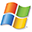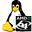ATOMS : Indirect Influences for Graphs details

# Indirect Influences for Graphs

Algorithms to calculate influences of graphs
Details
Version
1.52
A more recent valid version with binaries for Scilab 5.4 exists: 1.55
Author
Jorge Catumba
Owner Organization
U. Sergio Arboleda, Universidad Nacional de Colombia
Maintainer
Jorge Catumba
Categories
Dependency
Creation Date
February 6, 2012
Source created on
Scilab 5.4.x
Binaries available on
Scilab 5.4.x:Windows 64-bitWindows 32-bitLinux 64-bitLinux 32-bitmacOS
Install command
`--> atomsInstall("indinf")`
Report a bugDescription
```            Algorithms to calculate indirect influences and others for matrices. The
functions given with this package are:
* polyexpm - Function that returns the exponential matrix of a polynomial
matrix.
* polylogm - Function that returns the logarithm matrix of a polynomial
matrix.
* micmac - Function that applies the MICMAC method to a direct influences
matrix.
* pagerank - Function that applies the PageRank method to a direct influences
matrix.
* pwpIndInf - Function that applies the PWP method to a direct influences
matrix.
* heatkernel - Function that applies the Heat Kernel method to a direct
influences matrix.
* sum_matrix_blocks - Function that sums the blocks of a squared matrix.
* part_adj_mat - Function that calculates the adjcacency matrix of a partition
graph for a given graph.            ```Files (2)Source code archive
```
```OS-independent binary for Scilab 5.4.x
```Binary version
Automatically generated by the ATOMS compilation chain

```News (0)Comments (0)